NEET  >  Universal Law of Gravitation

# Universal Law of Gravitation Notes | Study Physics Class 11 - NEET

## Document Description: Universal Law of Gravitation for NEET 2022 is part of Physics Class 11 preparation. The notes and questions for Universal Law of Gravitation have been prepared according to the NEET exam syllabus. Information about Universal Law of Gravitation covers topics like Newton’s Law Of Universal Gravitation and Universal Law of Gravitation Example, for NEET 2022 Exam. Find important definitions, questions, notes, meanings, examples, exercises and tests below for Universal Law of Gravitation.

Introduction of Universal Law of Gravitation in English is available as part of our Physics Class 11 for NEET & Universal Law of Gravitation in Hindi for Physics Class 11 course. Download more important topics related with notes, lectures and mock test series for NEET Exam by signing up for free. NEET: Universal Law of Gravitation Notes | Study Physics Class 11 - NEET
 1 Crore+ students have signed up on EduRev. Have you?

Newton’s Law Of Universal Gravitation

Newton’s Law of Universal Gravitation states that every particle attracts every other particle in the universe with a force that is directly proportional to the product of the masses and inversely proportional to the square of the distance between them.

The universal gravitation equation thus takes the form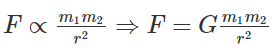Sir Isaac Newton put forward the universal law of gravitation in 1687 and used it to explain the observed motions of the planets and moons. In this article, let us familiarize ourselves with Newton’s Law of Universal Gravitation.

Universal Gravitation Equation

Newton’s conclusion about the magnitude of gravitational force is summarized symbolically as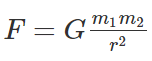where, F is the gravitational force between bodies, m1 is the mass of one of the objects, m2 is the mass of the second object, r is the distance between the centres of two objects, G is the universal gravitational constant.
The constant proportionality (G) in the above equation is known as the universal gravitation constant. Henry Cavendish experimentally determined the precise value of G. The value of G is found to be G = 6.673 x 10-11 N m2/kg2.

Gravitation Equation Vector Form

The universal gravitation equation is represented in vector form as follows: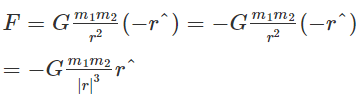where G is the gravitational constant and r is the unit vector from m1 to m2. The figure below shows the gravitational force on m1 due to m2 along with r where the vector r is (r2–r1).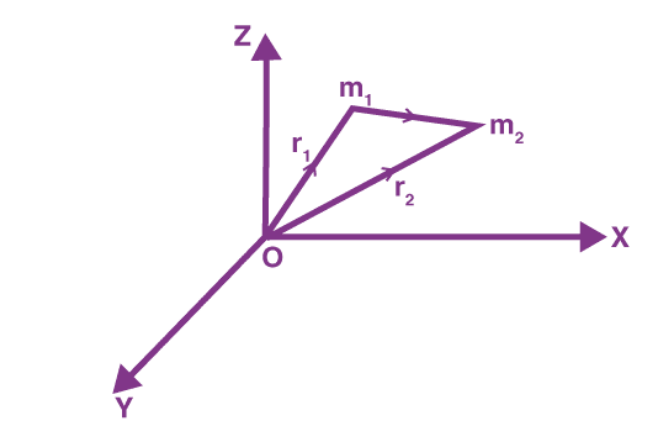If we have a collection of point masses, the force on any one of them is the vector sum of the gravitational forces exerted by the other point masses: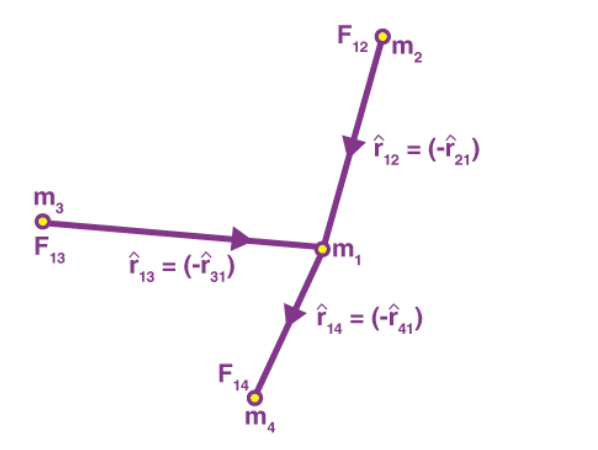The gravitational force on point mass m1 is the vector sum of the gravitational forces exerted by m2, m3 and m4. Therefore, the total force on m1 is given by: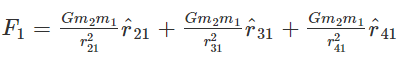Gravitational Constant
The value of the gravitational constant is extremely difficult to measure accurately. Henry Cavendish devised a clever apparatus for measuring the gravitational constant.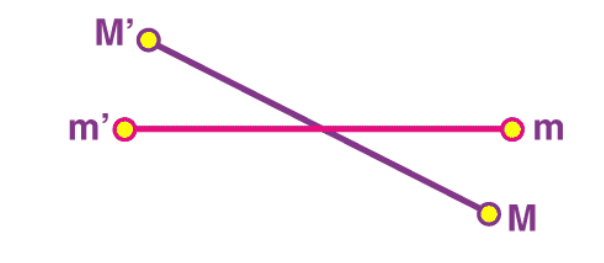The masses m and m’ are attached to either end of the beam as shown in the figure. The beam is attached to strong support with the help of a string. The string is tied to the middle of the beam allowing it to reach an equilibrium. Now, two large masses M’ and M are lowered next to them. The gravitational force between the two pairs of masses causes the string to twist such that the amount of twisting is just balanced by the gravitational force. The gravitational force can be measured by appropriate calibration. Since we know the value of masses and distances between them, the only unknown quantity is G in the universal law of gravitation. Thus, the value of G is calculated from the measured quantities.

Universal Gravitation Solved Example
Calculate the gravitational force of attraction between the Earth and a 70 kg man standing at a sea level, a distance of 6.38 x 106 m from the earth’s centre.
Solution:
Given:
m1 is the mass of the Earth which is equal to 5.98 x 1024 kg
m2 is the mass of the man which is equal to 70 kg
d = 6.38 x 106 m
The value of G = 6.673 x 10-11 N m2/kg2
Now substituting the values in the Gravitational force formula, we get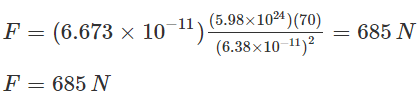Weight and the Gravitational Force
In Newton’s law of gravity, we noticed that the mass is a crucial quantity. We consider mass and weight to be the same, but they are related but are different in reality. Weight is the gravitational force exerted on an object of a certain mass. The object’s weight can be obtained by multiplying the mass m of the object by the acceleration due to gravity, g, at the Earth’s surface. The measured gravitational acceleration at the Earth’s surface is found to be about 980 cm/second/second.
The measure of how much material is in an object is known as mass, while weight is the measure of the gravitational force exerted on the material in a gravitational field; thus, mass and weight are proportional to each other acceleration due to gravity as the proportionality constant. Thus, it is observed that the mass is constant for a given object, but the weight depends on the location of the object. To better understand, let us consider the following example, say we transported an object of mass m to the surface of Neptune, the gravitational acceleration would change because the radius and mass of the Neptune both differ from those of the Earth. Thus, our object has mass m both on the surface of the Earth and on the surface of the Neptune, but it will weigh much more on the surface of Neptune because the gravitational acceleration there is 11.15 m/s2.

Universality of Gravity

Gravitation interactions not only exist between the earth and other objects, but it also exists between all objects with an intensity that is directly proportional to the product of their masses. The law of universal gravitation helps scientists study planetary orbits. The small perturbations in a planet’s elliptical motion can be easily explained owing to the fact that all objects exert gravitational influences on each other.

Why doesn’t’ the moon crash into the earth?

The forces of speed and gravity are what keeps the moon in constant orbit around the earth. The Moon seems to hover around in the sky, unaffected by gravity. However, the reason the Moon stays in orbit is precise because of gravity. In this video, clearly, understand why the moon doesn’t fall into the earth

Is the force of Gravity the same all over the Earth?

Gravity isn’t the same everywhere on earth. Gravity is slightly stronger over the places with more underground mass than places with less mass. NASA uses two spacecraft to measure the variation in the Earth’s gravity. These spacecraft are a part of the Gravity Recovery and Climate Experiment (GRACE) mission.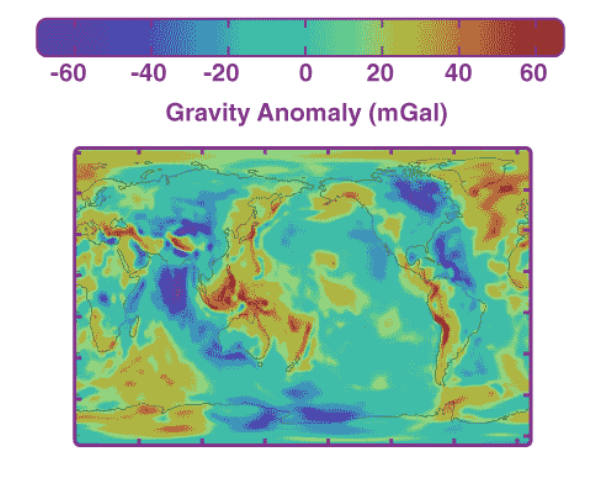Areas in blue have weaker gravity while areas in red have slightly stronger gravity.

The document Universal Law of Gravitation Notes | Study Physics Class 11 - NEET is a part of the NEET Course Physics Class 11.
All you need of NEET at this link: NEET

## Physics Class 11

126 videos|449 docs|210 tests
 Use Code STAYHOME200 and get INR 200 additional OFF

## Physics Class 11

126 videos|449 docs|210 tests

### How to Prepare for NEET

Read our guide to prepare for NEET which is created by Toppers & the best Teachers

Track your progress, build streaks, highlight & save important lessons and more!

,

,

,

,

,

,

,

,

,

,

,

,

,

,

,

,

,

,

,

,

,

;